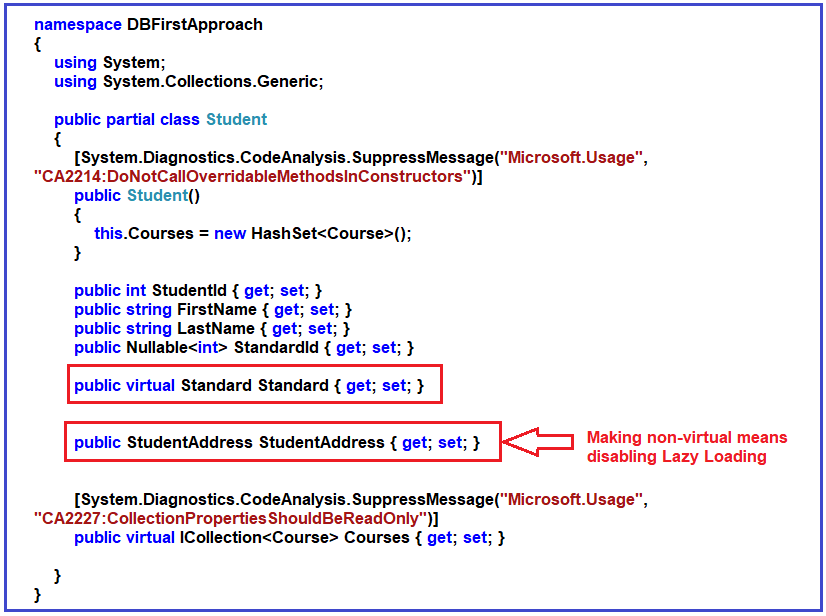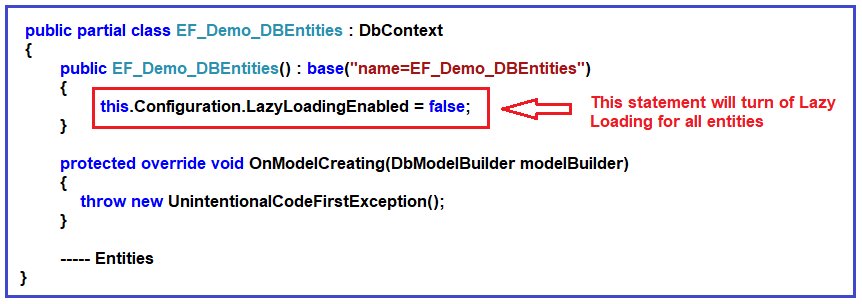Note: We are going to work with the same example that we created in our Introduction to Entity Framework Database First articlePlease read our introduction to Entity Framework Database First article before proceeding to this article.

Lazy Loading is the default behavior of Entity Framework. That means the related entities or child entities are loaded only when it is being accessed for the first time. That means in simple words we can say that Lazy loading simply delaying the loading of related entities until you specifically request for it.

Please have a look at the following Student entity which is generated by the Entity Framework. If you look at the Student class, then you will find a navigation property for the StudentAddress entity. At the same time, you will also find a navigation property for Standard and a collection navigation property for Courses.

```namespace DBFirstApproach
{
using System;
using System.Collections.Generic;

public partial class Student
{
[System.Diagnostics.CodeAnalysis.SuppressMessage("Microsoft.Usage", "CA2214:DoNotCallOverridableMethodsInConstructors")]
public Student()
{
this.Courses = new HashSet<Course>();
}

public int StudentId { get; set; }
public string FirstName { get; set; }
public string LastName { get; set; }
public Nullable<int> StandardId { get; set; }

public virtual Standard Standard { get; set; }
public virtual ICollection<Course> Courses { get; set; }
}
}
```

In the lazy loading, the context first loads the Student entity data from the database, then it will load the related entities when you access that navigation properties.

For example, when the below statement is executed, the context will only load the Student table. It will not load the related StudentAddress, Standard, or Courses table data.
Student student = context.Students.FirstOrDefault(std => std.StudentId == 1);

Once the below statement is executed, the Context will load the StudentAddress table data.

Similarly, when the below statement is executed, the Context will load the related Standard table data.
Standard standard = student.Standard;

##### The Complete Example is given below.
```using System;
using System.Linq;
namespace DBFirstApproach
{
class Program
{
static void Main(string[] args)
{
using (EF_Demo_DBEntities context = new EF_Demo_DBEntities())
{
Student student = context.Students.FirstOrDefault(std => std.StudentId == 1);
Console.WriteLine(\$"Firstname: {student.FirstName}, Lastname: {student.LastName}");

Standard standard = student.Standard;
Console.WriteLine(\$"StandardName: {standard.StandardName}, Description: {standard.Description}");

var courses = student.Courses;
foreach(var course in courses)
{
Console.WriteLine(\$"CourseName: {course.CourseName}");
}
}
}
}
}
```

The code shown above will result in four SQL queries.

First, it will fetch the particular student data by executing the below SQL Query when the (Student student = context.Students.FirstOrDefault(std => std.StudentId == 1);) statement is executed:

```SELECT TOP (1)
[Extent1].[StudentId] AS [StudentId],
[Extent1].[FirstName] AS [FirstName],
[Extent1].[LastName] AS [LastName],
[Extent1].[StandardId] AS [StandardId]
FROM [dbo].[Student] AS [Extent1]
WHERE 1 = [Extent1].[StudentId]
```

```exec sp_executesql N'SELECT
[Extent1].[StudentId] AS [StudentId],
[Extent1].[Mobile] AS [Mobile],
[Extent1].[Email] AS [Email]
WHERE [Extent1].[StudentId] = @EntityKeyValue1',N'@EntityKeyValue1 int',@EntityKeyValue1=1
```

When (Standard standard = student.Standard;) statement is executed, it will send the following SQL query to the database:

```exec sp_executesql N'SELECT
[Extent1].[StandardId] AS [StandardId],
[Extent1].[StandardName] AS [StandardName],
[Extent1].[Description] AS [Description]
FROM [dbo].[Standard] AS [Extent1]
WHERE [Extent1].[StandardId] = @EntityKeyValue1',N'@EntityKeyValue1 int',@EntityKeyValue1=1
```

When (var courses = student.Courses;) statement is executed, it will send the following SQL query to the database:

```exec sp_executesql N'SELECT
[Extent2].[CourseId] AS [CourseId],
[Extent2].[CourseName] AS [CourseName],
[Extent2].[TeacherId] AS [TeacherId]
FROM  [dbo].[StudentCourse] AS [Extent1]
INNER JOIN [dbo].[Course] AS [Extent2] ON [Extent1].[CourseId] = [Extent2].[CourseId]
WHERE [Extent1].[StudentId] = @EntityKeyValue1',N'@EntityKeyValue1 int',@EntityKeyValue1=1
```

We can disable lazy loading for a particular entity or a context (for all entities).

##### Turning Off for a particular entity:

To turn off lazy loading for a particular property, do not make it virtual. Lazy loading of the StudentAddress or Standard can be turned off by making the StudentAddress or Standard property non-virtual in the Student Entity as shown below.Please modify the auto-generated Student entity as shown below.

```namespace DBFirstApproach
{
using System;
using System.Collections.Generic;

public partial class Student
{
[System.Diagnostics.CodeAnalysis.SuppressMessage("Microsoft.Usage", "CA2214:DoNotCallOverridableMethodsInConstructors")]
public Student()
{
this.Courses = new HashSet<Course>();
}

public int StudentId { get; set; }
public string FirstName { get; set; }
public string LastName { get; set; }
public Nullable<int> StandardId { get; set; }

public virtual Standard Standard { get; set; }
public virtual ICollection<Course> Courses { get; set; }
}
}
```

```using System;
using System.Linq;
namespace DBFirstApproach
{
class Program
{
static void Main(string[] args)
{
using (EF_Demo_DBEntities context = new EF_Demo_DBEntities())
{
Student student = context.Students.FirstOrDefault(std => std.StudentId == 1);
Console.WriteLine(\$"Firstname: {student.FirstName}, Lastname: {student.LastName}");

{
}
else
{
}

}
}
}
}
```

Now, you can also verify the same using SQL Profiler and you will see only one SQL Statement is logged in the profiler. But if you access the Standard navigation property on the student object, then it will send an SQL query to the database and load the data as we have not disabled Lazy Loading for the Standard entity as shown below.

```using System;
using System.Linq;
namespace DBFirstApproach
{
class Program
{
static void Main(string[] args)
{
using (EF_Demo_DBEntities context = new EF_Demo_DBEntities())
{
Student student = context.Students.FirstOrDefault(std => std.StudentId == 1);
Console.WriteLine(\$"Firstname: {student.FirstName}, Lastname: {student.LastName}");

Standard standard = student.Standard;

if(standard == null)
{
}
else
{
Console.WriteLine(\$"StandardName {standard.StandardName}, Description {standard.Description}");
}

}
}
}
}
```